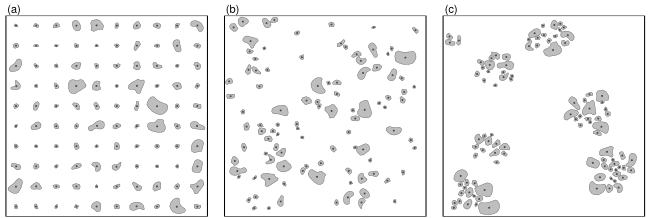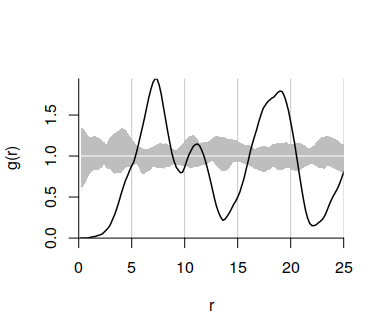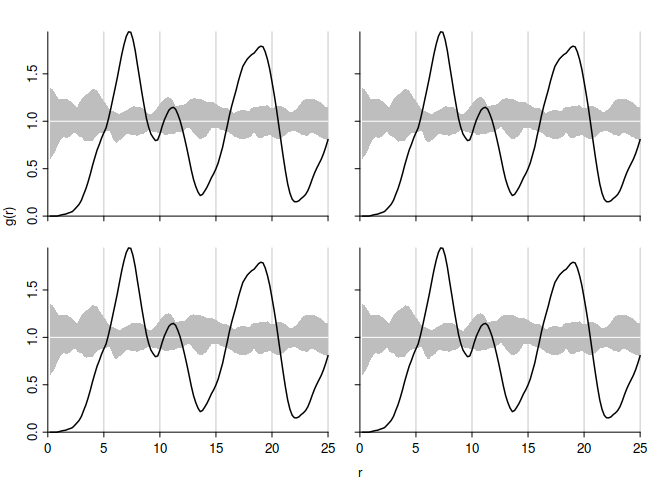# Introduction to apcf

#### 2020-02-20

The apcf package provides methods to analyse patterns of objects of finite size and irregular shape using the adapted Pair-Correlation Function (PCF) as proposed by Nuske et al. (2009).

## Background

The method suggested by Nuske et al. (2009) requires a certain number of null models for correcting the biased PCF and constructing a pointwise critical envelope. Null models are constructed by randomly moving (shift and rotation) the objects within the study area.

The alpha level of the pointwise critical envelope is $$\alpha = {n\_rank * 2} / {n\_sim + 1}$$ according to (Besag and Diggle 1977, Buckland 1984, Stoyan and Stoyan, 1994).

For the edge correction (based on Ripley, 1981) a buffer with buffer distance $$r_{ij}$$ is constructed around the object i for each pair of objects i and j. The object j is then weighted by the inverse of the proportion $$p_{ij}$$ of the buffer perimeter being within the study area.

Being a density function the frequently used Epanechnikov kernel (Silverman, 1986; Stoyan and Stoyan, 1994) is used for smoothing the PCF. The smoothing is controlled by the bandwith parameter $$\delta$$ and the step size r. Penttinen et al. (1992) and Stoyan and Stoyan (1994) suggest to set c aka stoyan-parameter of $$c / {\sqrt{\lambda}}$$ between 0.1 and 0.2 with $$\lambda$$ being the intensity of the pattern.

To separate the computationally intensive part (randomization of the objects and the calculation of the proportion of the buffer inside the study area) from the smoothing of the PCF we use a two step approach:

• calculate distances and buffer fractions for original and randomized patterns
• turn the distances and buffer fractions into a PCF together with a pointwise critical envelope

## Example Data

To present the workflow we make use of the simulated patterns presented in Nuske et al. (2009). The Shapefiles are part of the package apcf and documented as ?simulated patternsSimulated patterns (a: regular, b: random, c: clustered)

## From Pattern to Distances

pat2dists() calculates distances between all object of a pattern closer than max_dist and determines the fraction of a buffer with distance dist inside the study area (needed for edge correction). It randomizes the original pattern to generate n_sim null models used for correcting the biased PCF and constructing an envelope.

It returns an object of class dists containing a data.frame with the columns sim, dist, and prop with an indicator of the model run (0:n_sim), distances between the objects of the patterns, and the proportion of a buffer with distance dist inside the study area. The size of the study area, the total number of objects, and the maximum distance are passed along as well.

dists <- pat2dists(area=system.file("shapes/sim_area.shp", package="apcf"),
pattern=system.file("shapes/sim_pat_reg.shp", package="apcf"),
max_dist=25, n_sim=9, verbose=FALSE)
##   sim     dist     ratio
## 1   0 24.64805 0.9240050
## 2   0 17.15525 1.0000000
## 3   0 20.22547 1.0000000
## 4   0 20.04375 1.0000000
## 5   0 24.13931 0.9517569
## 6   0 19.67672 1.0000000

## From Distances to PCF

dists2pcf() estimates the adapted pair correlation function of a pattern of polygons together with a pointwise critical envelope using kernel methods based on distances between objects.

It returns an object of class fv_pcf containing the function values of the PCF and the pointwise critical envelope. The number of null models, the rank of envelope value among the n_sim values and the bandwith/stoyan parameter are passed along as well.

pcf <- dists2pcf(dists, r=0.2, r_max=25, stoyan=0.15, n_rank=1)
## PCF with pointwise critical envelopes
## Obtained from 9 simulations
## Edge correction: "Ripley"
## Alternative: "two.sided"
## Significance level of pointwise Monte Carlo test: 0.2
##
##     r           g       lwr      upr
## 1 0.2 0.000000000 0.5992990 1.351866
## 2 0.4 0.000000000 0.6310871 1.339156
## 3 0.6 0.000000000 0.6659674 1.306272
## 4 0.8 0.000000000 0.7205283 1.266185
## 5 1.0 0.005371333 0.7753014 1.225430
## 6 1.2 0.012187492 0.8107138 1.234002

## Plotting the PCF

plot.fv_pcf() is a plot method for the class fv_pcf. It draws a pair correlation function and a pointwise critical envelope if available.

plot(pcf)# a panel of four plots
op <- par(mfrow=c(2,2), oma=c(3,3,0,0), mar=c(0,0,2,2),
mgp=c(2,0.5,0), tcl=-0.3)
plot(pcf, xaxis='t', yaxis='o', ann=FALSE)
plot(pcf, xaxis='t', yaxis='t', ann=FALSE)
plot(pcf, xaxis='o', yaxis='o', ann=FALSE)
plot(pcf, xaxis='o', yaxis='t')
par(op)## Technical Details

The adapted pair-corelation functions was original implemented in the Geodatabase PostGIS. PostGIS offers all necessary methods and is easy to handle knowing databases and SQL. The need to create lots of temporary tables and countless transformations of geodata from WKB stored in the table to the GEOS format for all geometric operations rendered the process quite slow.

In this package the geometric operations (measuring distances between objects, buffers, intersects, and randomly moving objects) are carried out by GEOS. The geodata is read and transformed to GEOS format only once (thanks to GDAL/OGR all conceivable formats are understood). All further manipulations are within the GEOS world. The usage of these two libaries was made possible by Rcpp.